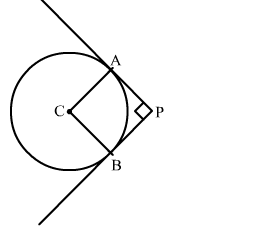# A solid cone of base radius 10 cm is cut into two parts

Question:

A solid cone of base radius 10 cm is cut into two parts through the mid-point of its height, by a plane parallel to its base. Find the ratio in the volumes of two parts of the cone.

Solution:Let the height of the cone be H.
Now, the cone is divided into two parts by the parallel plane

∴ OC = CAH2

Now, In ∆OCD and OAB

∠OCD = OAB          (Corresponding angles)

∠ODC = OBA          (Corresponding angles)

By AA-similarity criterion ∆OCD ∼ ∆OAB

$\therefore \frac{\mathrm{CD}}{\mathrm{AB}}=\frac{\mathrm{OC}}{\mathrm{OA}}$

$\Rightarrow \frac{\mathrm{CD}}{10}=\frac{H}{2 \times H}$

$\Rightarrow \mathrm{CD}=5 \mathrm{~cm}$

$\frac{\text { Volume of first part }}{\text { Volume of second part }}=\frac{\frac{1}{3} \pi(\mathrm{CD})^{2}(\mathrm{OC})}{\frac{1}{3} \pi \mathrm{CA}\left[(\mathrm{AB})^{2}+(\mathrm{AB})(\mathrm{CD})+\mathrm{CD}^{2}\right]}$

$=\frac{(5)^{2}}{\left[(10)^{2}+(10)(5)+5^{2}\right]}$

$=\frac{25}{100+50+25}$

$=\frac{25}{175}$

$=\frac{1}{7}$